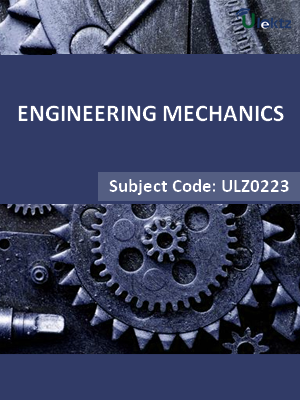•My WalletMy Order
•My Profile
•My Connections
•My Books
•My Videos
•My Tests
•My Calender
•My Messages
•My Shopping Cart
•My Orders
•Account Settings
•Help

# Book Details# Engg. Mechanics (Plane curvilinear motion of particles)

 Course Code : ULZ0223 Author : uLektz University : General for All University Regulation : 2017 Categories : Mechanical Format :ePUB3 (DRM Protected) Type : eBook

FREE

Description :Engg. Mechanics (Plane curvilinear motion of particles) of ULZ0223 covers the latest syllabus prescribed by General for All University for regulation 2017. Author: uLektz, Published by uLektz Learning Solutions Private Limited.

Note : No printed book. Only ebook. Access eBook using uLektz apps for Android, iOS and Windows Desktop PC.

##### Topics
###### MODULE - I IMPORTANCE OF MECHANICS IN ENGINEERING

1.1 Importance of Mechanics in engineering; Introduction to Statics;Concept of Particle and Rigid Body

1.2 Types of forces: collinear, concurrent, parallel, concentrated, distributed;Vector and scalar quantities; Force is a vector;Transmissibility of a force (sliding vector)

1.3 Introduction to Vector Algebra;Parallelogram law;Addition and subtraction of vectors;Lami’s theorem;Free vector; Bound vector;Free vector; Bound vector

1.4 Representation of forces in terms of i,j,k, Cross product and Dot product and their applications.

1.5 Two dimensional force system; Resolution of forces,Moment; Varignon’s theorem; Couple, Resolution of a coplanar force by its equivalent force-couple system; Resultant of forces

###### MODULE - II CONCEPT OF EQUILIBRIUM OF FORCES IN TWO DIMENSIONS AND FRICTION

2.1 Concept and Equilibrium of forces in two dimensions,Free body concept and diagram,Equations of equilibrium.

2.2 Concept of Friction, Laws of Coulomb friction, Angle of Repose,Coefficient of friction

###### MODULE - III CONCEPT OF SIMPLE STRESSES AND STRAINS AND DISTRIBUTED FORCE, MOMENTS OF INERTIA

3.1 Distributed Force: Centroid and Centre of Gravity;Centroids of a triangle, circular sector, quadralateral, composite areas consisting of above figures.

3.2 Moments of inertia: MI of plane figure with respect to an axis in its plane,MI of plane figure with respect to an axis perpendicular to the plane of the figure;

3.3 Parallel axis theorem, Mass moment of inertia of symmetrical bodies, e.g. cylinder, sphere, cone.

3.4 Concept of simple stresses and strains: Normal stress, Shear stress, Bearing stress, Normal strain, Hooke’s law; Poisson’s ratio

3.5 Stress-strain diagram of ductile and brittle materials; Elastic limit; Ultimate stress; Yielding; Modulus of elasticity,Factor of safety.

###### MODULE - IV INTRODUCTION TO DYNAMICS AND PLANE CURVILINEAR MOTION OF PARTICLES

4.1 Introduction to Dynamics: Kinematics and Kinetics,Newton’s laws of motion,Law of gravitation & acceleration due to gravity, Rectilinear motion of particles

4.2 Determination of position, velocity and acceleration under uniform and non-uniformly accelerated rectilinear motion; construction of x-t, v-t and a-t graphs.

4.3 Plane curvilinear motion of particles: Rectangular components (Projectile motion); Normal and tangential components (circular motion).

###### MODULE - V KINETICS OF PARTICLES

5.1 Kinetics of particles: Newton’s second law,Equation of motion,D.Alembert’s principle and free body diagram

5.2 Principle of work and energy, Principle of conservation of energy, Power and efficiency